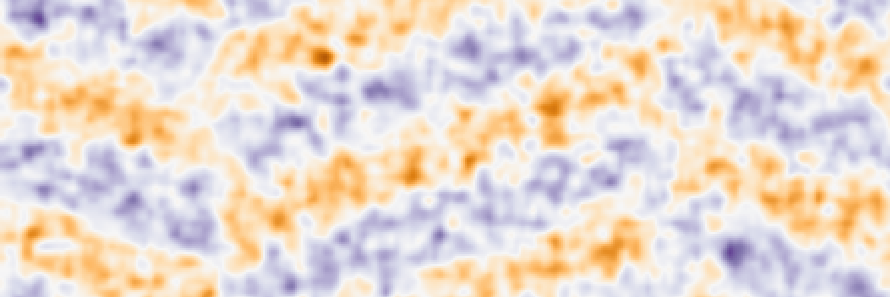# MM23 - Simultaneous ReconstructionsSimultaneously-reconstructed magnetic potential of antiferromagnetically ordered oxygen-terminated twin boundaries in NiO.

Georgios Varnavides, Stephanie Ribet, Reed Yalisove, Joel Moore, Colin Ophus, Mary Scott.

#### Abstract

While the observation of magnetic structure with nanometer spatial resolution has been successfully achieved with various transmission electron microscopy (TEM) techniques such as Lorentz TEM, off-axis electron holography, differential phase contrast (DPC), and more recently, Lorentz scanning TEM, atomic-scale imaging of magnetization remains challenging. This can be traced back to the extremely small phase-shifts acquired by the electron beam due to the magnetic vector potential, $$\boldsymbol{A}(x,y,z)$$, compared to those acquired due to the electrostatic potential of the sample, $$V(x,y,z)$$:

$\phi(x,y) = \phi_e(x,y) + \phi_m(x,y) = \sigma \int V(x,y,z) dz - \frac{e}{\hbar} \int A_z(x,y,z) dz,$

where $$\sigma$$ is the interaction parameter, $$e$$ is the elementary charge, and $$\hbar$$ is the reduced Planck’s constant.

Recent studies have addressed this inherent signal-to-noise challenge via kernel-filtering and unit-cell averaging of DPC measurements, centre-of-mass and principal component analysis, and more recently, Fourier-filtering of ptychographically-reconstructed phase images. While these techniques enable atomic-scale imaging of magnetization for the studied systems, they require prior knowledge of the magnetic ordering in the system and are unlikely to generalize well to non-periodic systems.

In this work, we introduce a novel ptychographic algorithm to simultaneously reconstruct the electrostatic and magnetic vector potential from two sets of diffraction intensity measurements, in which the sign of the magnetic contribution to the phase is reversed, e.g., by flipping the sample 180 degrees. Separating the electrostatic and magnetic contributions to the retrieved phase shifts from two sets of measurements is routinely performed in off-axis electron holography:

$\phi_e = \frac{1}{2}\left(\phi^{\rightarrow}+\phi^{\leftarrow}\right) \qquad, \qquad \phi_m = \frac{1}{2}\left( \phi^{\rightarrow} - \phi^{\leftarrow}\right),$

and indeed such a “background-subtraction” technique can be applied to ptychographic reconstructions mutatis mutandis. However, the procedure is prone to amplify noise in the magnetic potential phase by subtracting two potentially noisy reconstructions.

By contrast, our algorithm, which is implemented in the open-source 4D-STEM processing and analysis toolkit py4DSTEM, uses a physically inspired forward model for the overlap projection which distinguishes between the “forward” and “reverse” measurement directions by conjugating the complex magnetic potential object. This enables high signal-to-noise reconstructions, while also allowing for different regularization constraints on the electrostatic and magnetic potential objects, e.g., using a multislice propagator for the electrostatic potential object while assuming the magnetic potential is a single-slice pure-phase object.

We illustrate the performance of the algorithm on simulated datasets for antiferromagnetic (AFM) NiO lattices, chosen due to its high Néel temperature of 523K and insulating collinear antiferromagnetic phase of alternating ferromagnetic (111) planes. Figure 1 shows the electrostatic and magnetic contributions to the phase along the beam direction for bulk antiferromagnetic NiO, calculated using collinear density functional theory. Due to the orders of magnitude difference between the two scattering signals, the “background subtraction” reconstruction for a simulated dataset with finite dose is noisy and quantitatively incorrect, recovering a lower phase shift than the simultaneous reconstruction algorithm.

To further demonstrate the robustness of the reconstruction algorithm on non-periodic structures, we consider two types of oxygen-terminated twin boundaries (TB) in NiO with different antiferromagnetic ordering (Figure 2). First, note that while the twin-boundary is immediately obvious in the reconstructed electrostatic potential (or equivalently in HRTEM/STEM imaging), certain antiferromagnetic orderings across the twin boundary give similar magnetic phase contributions as bulk NiO (in our chosen zone-axis). Conversely, conventional imaging techniques cannot differentiate between different magnetic orderings across the twin boundary, in contrast to the simultaneous reconstruction algorithm.

Finally, we comment on the choice of experimental design parameters, such as convergence angle and sample thickness, as-well as reconstruction parameters, such as low-pass filtering and various projection-set algorithms, as means to amplify the magnetic signal, while sacrificing resolution in the electrostatic potential.